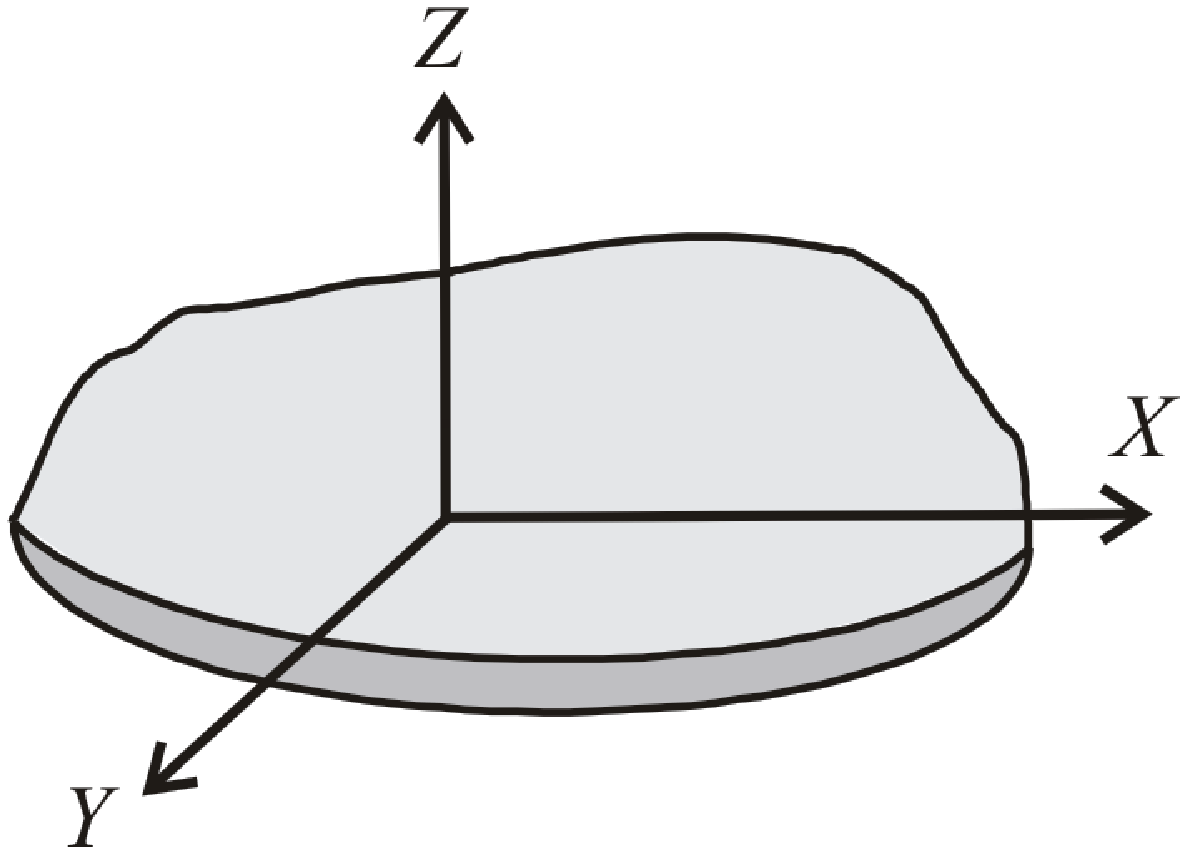BodhiAI
Oct. 31, 2019

#### Perpendicular Axis Theorems:

Theorem of perpendicular axes:

According to this theorem, the sum of moment of inertia of a plane lamina about two mutually perpendicular axes lying in its plane is equal to its moment of inertia about an axis perpendicular to the plane of lamina and passing through the point of intersection of the first two axes.Iz = Iz + Iy

Example : Moment of inertia of a disc about an axis through its centre of mass and perpendicular to its plane is MR2/2 , so if the disc is in x–y plane, then by theorem of perpendicular axesi.e. Iz = Ix + Iy

where•